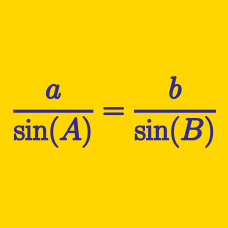Geometry

# Solving Triangles: Level 4 Challenges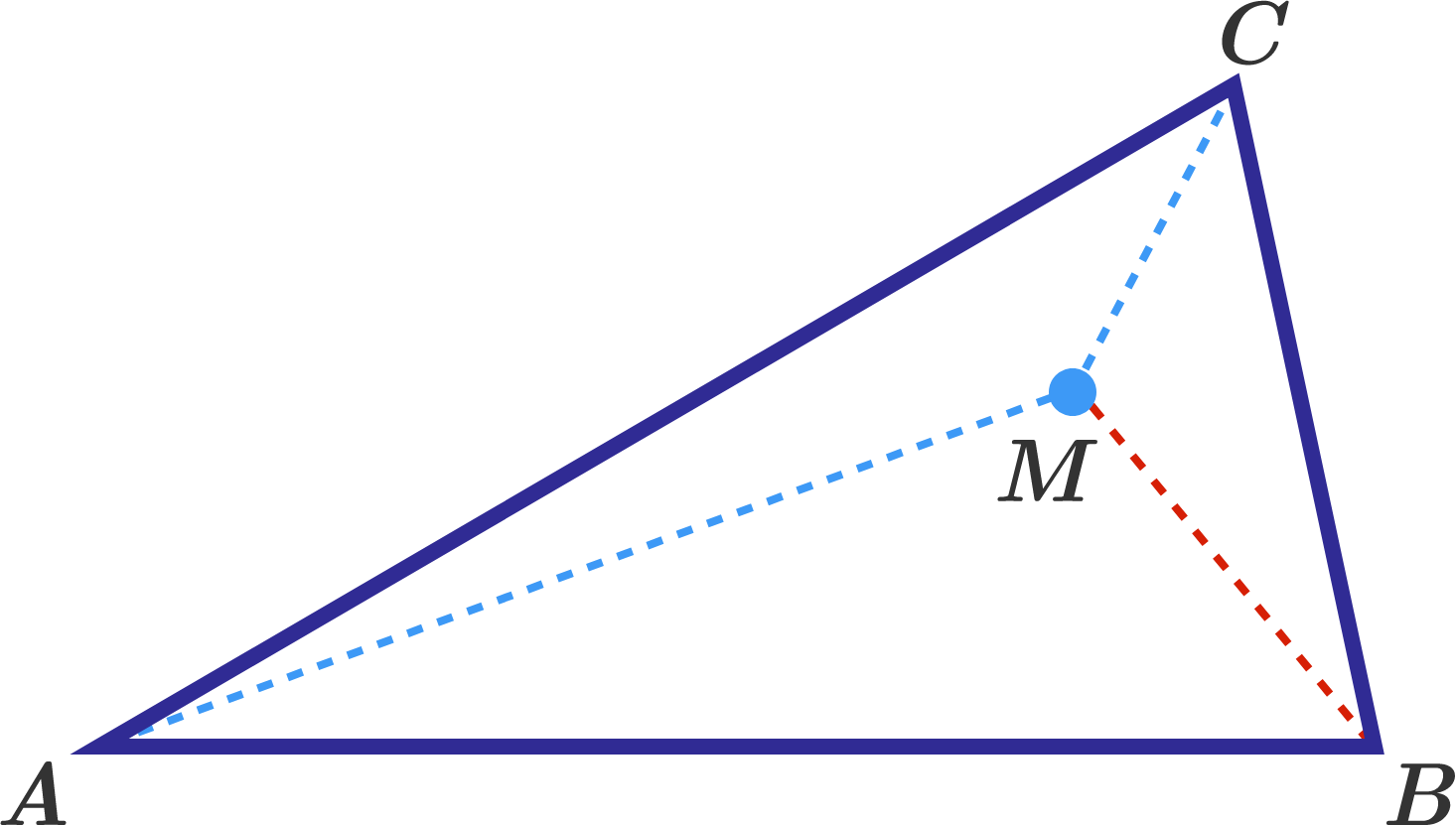In a triangle $ABC$, the measures of the angles are $\angle A=30^\circ,$ $\angle B=80^\circ.$ Point $M$ lies inside the triangle, such that $\angle MAC = 10^\circ,$ $\angle MCA = 30^\circ.$ What is the measure (in degrees) of $\angle BMC?$

Triangle $ABC$ is isosceles with $AC = BC$ and $\angle ACB = 106^\circ$ Point $M$ is inside the triangle so that $\angle MAC = 7^\circ$ and $\angle MCA = 23^\circ$. Find the measure of $\angle CMB$ in degrees.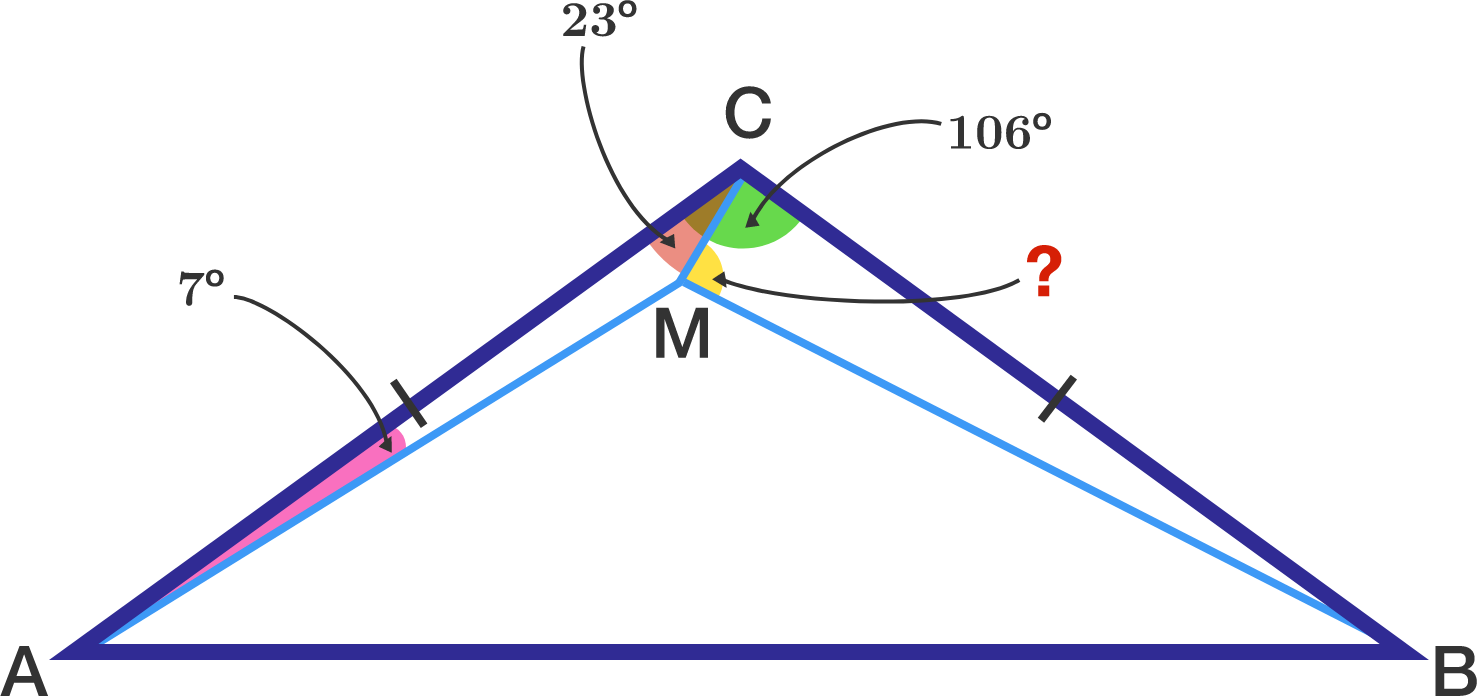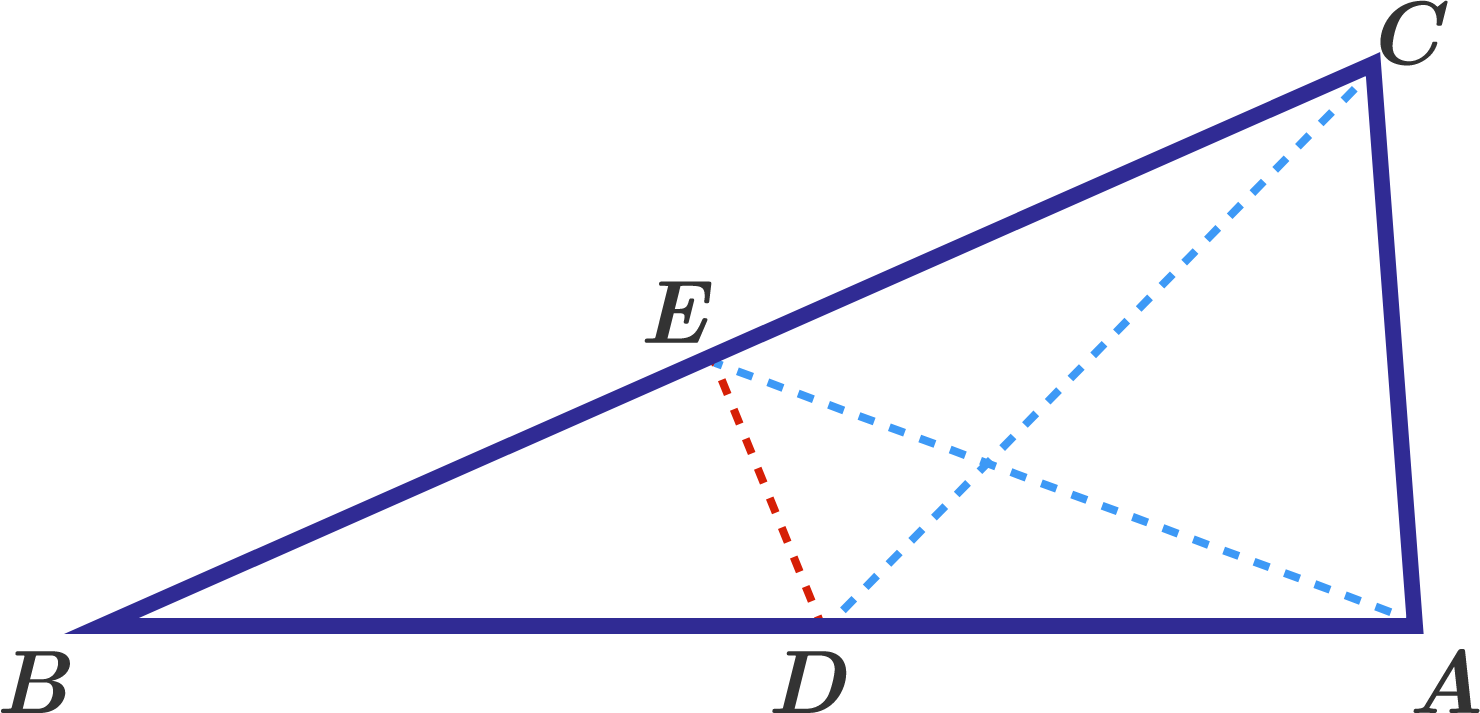In a triangle $ABC$ $\angle A =84^\circ,$ $\angle C=78^\circ.$ Points $D$ and $E$ are taken on the sides $AB$ and $BC,$ so that $\angle ACD =48^\circ,$ $\angle CAE =63^\circ.$ What is the measure (in degrees) of $\angle CDE$ ?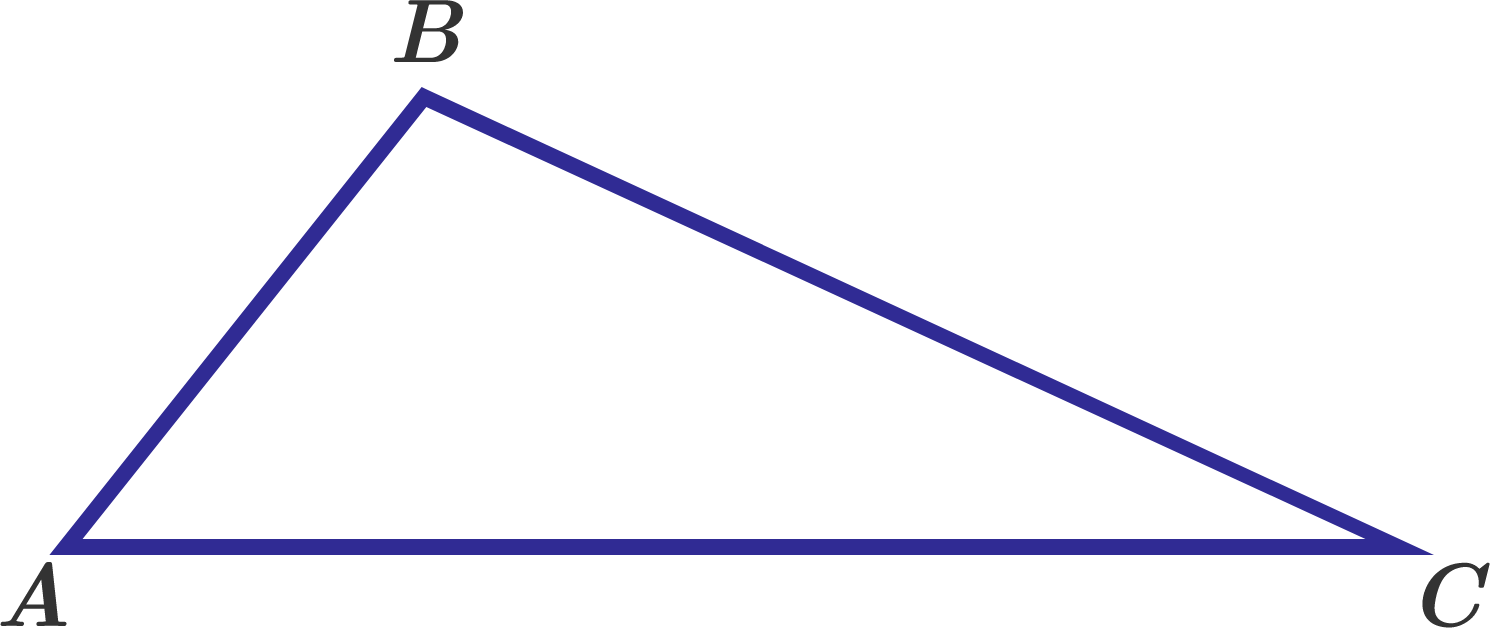In triangle $ABC$, $\cos A : \cos B : \cos C = 2 : 9 : 12$.

If $\sin A : \sin B : \sin C = p : q : r$, where $p$, $q$ and $r$ are coprime positive integers, find $pqr-(p+q+r)$.

Triangle $ABC$ has area equal to $\dfrac {90 \sqrt{3}}{4}$ and perimeter equal to $30.$ Also, one of its angles is equal to $60^\circ.$ What is the product of the sides of $ABC?$

×